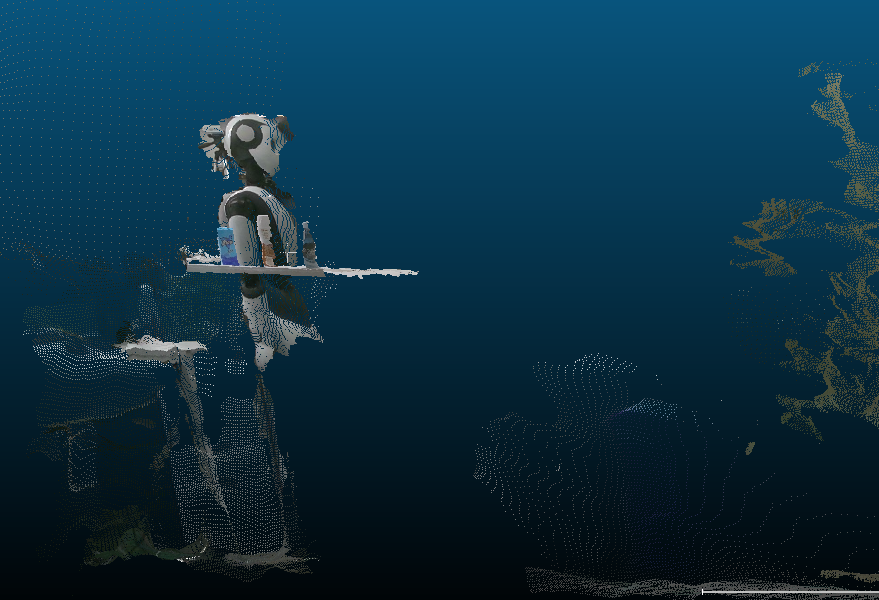# 基於Python深度圖生成3D點雲

theme: channing-cyan highlight: agate

# 二維RGB影象

```python [ [[r,g,b],[r,g,b],[r,g,b],[r,g,b]], [[r,g,b],[r,g,b],[r,g,b],[r,g,b]], ...

] ```

# 成像原理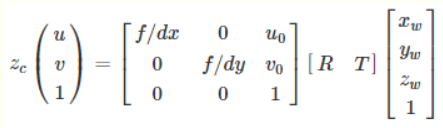其中u,v為二維平面上的任意點座標，1為深度，Zc就是咱們的二維平面上的點。

# 資料準備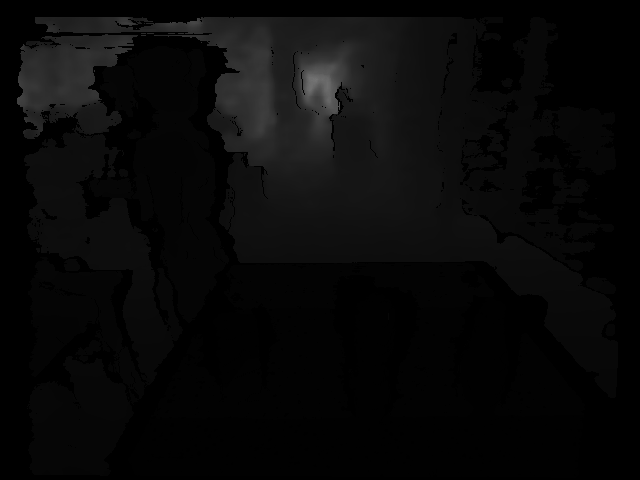# 圖片載入

```python

``````def __init__(self, rgb_file, depth_file, save_ply, camera_intrinsics=[784.0, 779.0, 649.0, 405.0]):
self.rgb_file = rgb_file
self.depth_file = depth_file
self.save_ply = save_ply

self.width = self.rgb.shape
self.height = self.rgb.shape

self.camera_intrinsics = camera_intrinsics
self.depth_scale = 1000
``````

``` 下面的這個東西，就是咱們公式當中的u0,v0,dx,dy

```python camera_intrinsics=[784.0, 779.0, 649.0, 405.0]``` 這個要注意的是，這個玩意呢，是不同的裝置相機有不同的引數，要根據自己的設定。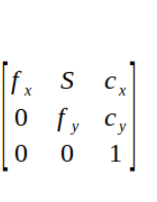```python self.depth_scale = 1000```

# 演算法實現

```python

``````    depth = np.asarray(self.depth, dtype=np.uint16).T
# depth[depth==65535]=0
self.Z = depth / self.depth_scale
fx, fy, cx, cy = self.camera_intrinsics

X = np.zeros((self.width, self.height))
Y = np.zeros((self.width, self.height))
for i in range(self.width):
X[i, :] = np.full(X.shape, i)

self.X = ((X - cx / 2) * self.Z) / fx
for i in range(self.height):
Y[:, i] = np.full(Y.shape, i)
self.Y = ((Y - cy / 2) * self.Z) / fy
``````

```

# 生成點雲

```python data_ply = np.zeros((6, self.width * self.height)) data_ply = self.X.T.reshape(-1) data_ply = -self.Y.T.reshape(-1) data_ply = -self.Z.T.reshape(-1) img = np.array(self.rgb, dtype=np.uint8) data_ply = img[:, :, 0:1].reshape(-1) data_ply = img[:, :, 1:2].reshape(-1) data_ply = img[:, :, 2:3].reshape(-1) self.data_ply = data_ply t2 = time.time() print('calcualte 3d point cloud Done.', t2 - t1)```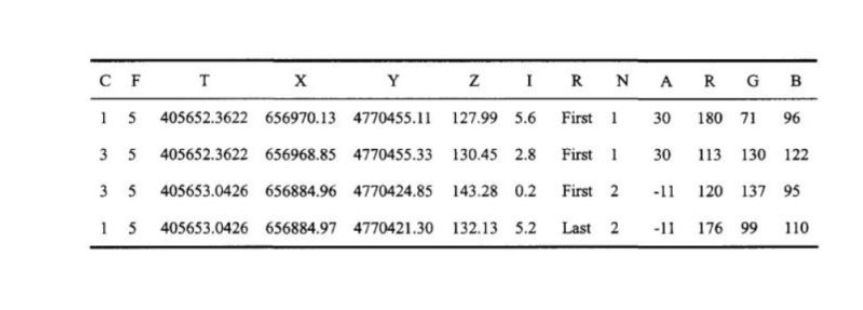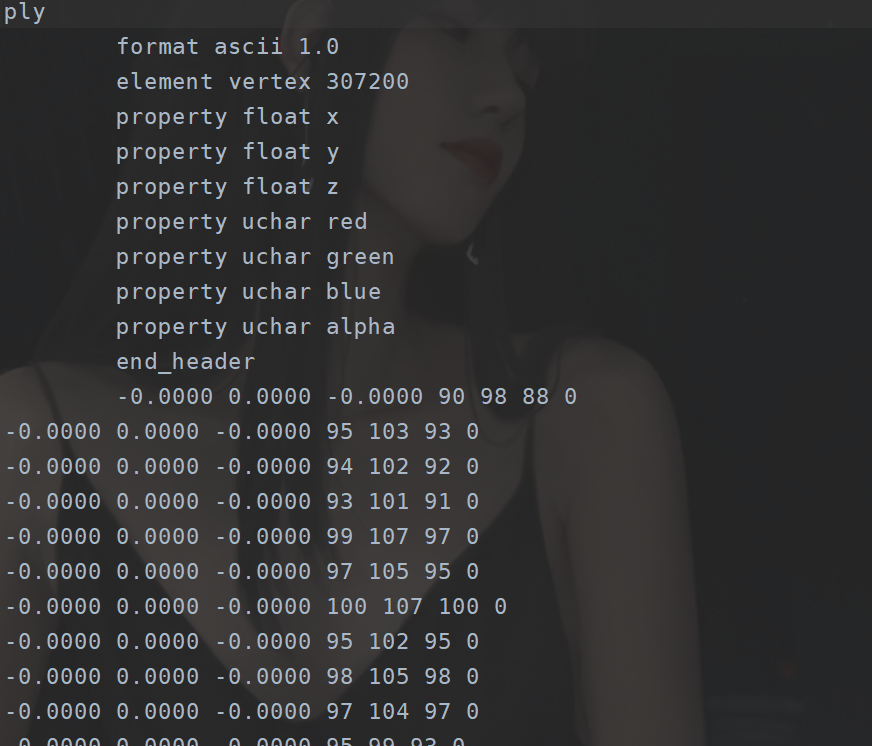```python start = time.time() float_formatter = lambda x: "%.4f" % x points = [] for i in self.data_ply.T: points.append("{} {} {} {} {} {} 0\n".format (float_formatter(i), float_formatter(i), float_formatter(i), int(i), int(i), int(i)))

``````    file = open(self.save_ply, "w")
file.write('''ply
format ascii 1.0
element vertex %d
property float x
property float y
property float z
property uchar red
property uchar green
property uchar blue
property uchar alpha
%s
''' % (len(points), "".join(points)))
file.close()

end = time.time()
print("Write into .ply file Done.", end - start)
``````

```

# 完整程式碼

```python import cv2 import numpy as np import open3d as o3d

import time

class point_cloud_generator():

``````def __init__(self, rgb_file, depth_file, save_ply, camera_intrinsics=[784.0, 779.0, 649.0, 405.0]):
self.rgb_file = rgb_file
self.depth_file = depth_file
self.save_ply = save_ply

self.width = self.rgb.shape
self.height = self.rgb.shape

self.camera_intrinsics = camera_intrinsics
self.depth_scale = 1000

def compute(self):
t1 = time.time()

depth = np.asarray(self.depth, dtype=np.uint16).T
# depth[depth==65535]=0
self.Z = depth / self.depth_scale
fx, fy, cx, cy = self.camera_intrinsics

X = np.zeros((self.width, self.height))
Y = np.zeros((self.width, self.height))
for i in range(self.width):
X[i, :] = np.full(X.shape, i)

self.X = ((X - cx / 2) * self.Z) / fx
for i in range(self.height):
Y[:, i] = np.full(Y.shape, i)
self.Y = ((Y - cy / 2) * self.Z) / fy

data_ply = np.zeros((6, self.width * self.height))
data_ply = self.X.T.reshape(-1)
data_ply = -self.Y.T.reshape(-1)
data_ply = -self.Z.T.reshape(-1)
img = np.array(self.rgb, dtype=np.uint8)
data_ply = img[:, :, 0:1].reshape(-1)
data_ply = img[:, :, 1:2].reshape(-1)
data_ply = img[:, :, 2:3].reshape(-1)
self.data_ply = data_ply
t2 = time.time()
print('calcualte 3d point cloud Done.', t2 - t1)

def write_ply(self):
start = time.time()
float_formatter = lambda x: "%.4f" % x
points = []
for i in self.data_ply.T:
points.append("{} {} {} {} {} {} 0\n".format
(float_formatter(i), float_formatter(i), float_formatter(i),
int(i), int(i), int(i)))

file = open(self.save_ply, "w")
file.write('''ply
format ascii 1.0
element vertex %d
property float x
property float y
property float z
property uchar red
property uchar green
property uchar blue
property uchar alpha
%s
''' % (len(points), "".join(points)))
file.close()

end = time.time()
print("Write into .ply file Done.", end - start)

def show_point_cloud(self):
``` 效果如下：